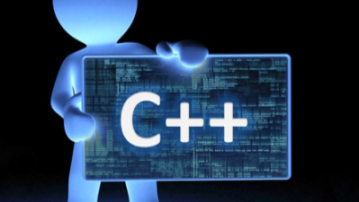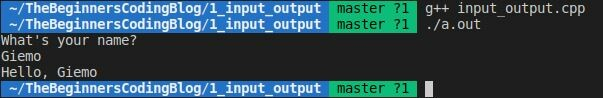# C++ Basics - [Part 2] Input/Output

By Giemo | The Beginners Coding Blog | 24 Feb 2021

Before we start with this lesson, I recommend adding your code to GitHub. It's free, easy to use and will help you in the long run. If you never used GitHub before, have a look at this guide, which will help you setting up a GitHub repository and get started.

In the previous lesson we looked at how we can create a terminal output. In this lesson, we will explore how that works and how we can handle user inputs in our code.

#### Output

As mentioned in the last lesson

``std::cout << "This text will be displayed in the terminal." << std::endl;``

will create an output on the terminal. This line of code can be split into the three important parts:

1) std::cout

If we want to print something to the terminal, there are a couple of ways to do so, but the easiest, most C++ way is to use std::cout. So if we want to write something to the terminal, we will always start with std::cout. This creates a buffer that will store the text which later is written to the terminal.

2) <<

This is the insertion operator. It signals the compiler that whatever follows gets inserted into the buffer above mentioned buffer. It is possible to have multiple insertions in one std::cout call. The following line will give the same output as the above code:

``std::cout << "This text will be " << "displayed in the terminal." << std::endl;``

3) " "

The most important part of creating an output is the output message. If we want to have a fixed text written to the terminal, we need to put it in quotes. Along this series we will encounter more ways to dynamically create terminal outputs, where quotes are not necessary anymore.

4) std::endl

To tell the compiler we are done constructing the output and are ready to send it to the terminal we use std::endl. Not only does this print the constructed message to the terminal, it also jumps the terminal into the next line. After the std::endl we of course need to add a semicolon, as we have to do for all instructions.

#### Input

Getting an input through the terminal is similar to how we create an output. Just by analyzing the bellow code, we can see some similarities:

``````std::string text;
std::cin >> text;``````

We will again break it down into the key components:

1) std::string text;

Before we can receive an input through the terminal, we need to have a variable, where we store the input we receive. Variables and Datatypes are going to be part of the next lesson, for now it is enough to know that text is a variable that we use to store the terminal input.

2) std::cin

Similar to the output, we need to tell the compiler that we want to receive an input through the terminal. Therefore we start this instruction with std::cin.

3) >> text

Because we now want to write the input that we received into text we need to use >> instead of <<. Before we used the insertion operator to insert text into a buffer, now we use the extraction operator to extract text out of the std::cin buffer. Since we are extracting the text, we need to store it somewhere, that's where the text variable comes into place std::cin >> text; stores the input we receive from the terminal in the text variable.

#### Bringing it all together

Let's write a short program, that utilizes the input and output functionality and quickly explain the steps on the go. Therefore we will use comments in our code. A comment in C++ starts with // and whatever follows gets ignored by the compiler completely.

``````// Include iostream to get access to std::cout, std::cin, std::endl and std::string
#include <iostream>

int main()
{
// Create a simple console output
std::cout << "What's your name?" << std::endl;

// Create a variable, where we store the input, that we're about to receive
std::string text;

// Request user input and store it in the variable called text
std::cin >> text;

// Create a console output using the user input that was stored in the variable text
std::cout << "Hello, " << text << std::endl;

return 0;
}``````

When we execute the code, our terminal should look something like this:Try different inputs and outputs to get a feel for how it works. If you have any question, stumble upon problems or want to give feedback on this lesson, feel free to drop a comment.

You can find the code of every lesson on GitHub.The Beginners Coding Blog

I am a software engineer with multiple years of excessive C++ experience. In this blog I will introduce you to the world of programming from scratch using the programming language C++. If you like to program, but don't know where to start, this blog will help you to get started.Send a \$0.01 microtip in crypto to the author, and earn yourself as you read!

20% to author / 80% to me.
We pay the tips from our rewards pool.